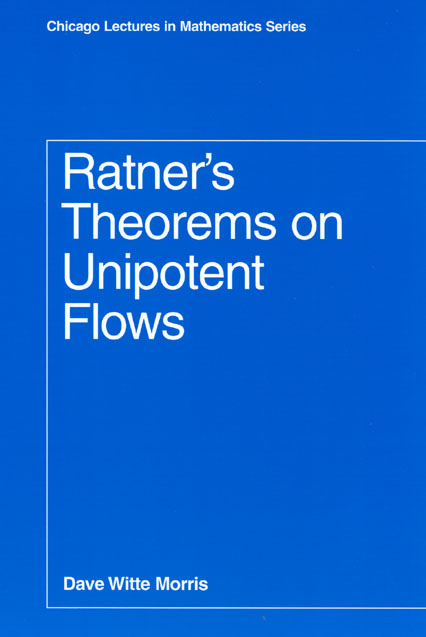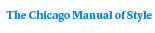# Ratner’s Theorems on Unipotent Flows

Dave Witte Morris203 pages | 6 x 9 | © 2005
Paper \$30.00 ISBN: 9780226539843 Published August 2005
The theorems of Berkeley mathematician Marina Ratner have guided key advances in the understanding of dynamical systems. Unipotent flows are well-behaved dynamical systems, and Ratner has shown that the closure of every orbit for such a flow is of a simple algebraic or geometric form. In Ratner’s Theorems on Unipotent Flows, Dave Witte Morris provides both an elementary introduction to these theorems and an account of the proof of Ratner’s measure classification theorem.

A collection of lecture notes aimed at graduate students, the first four chapters of Ratner’s Theorems on Unipotent Flows can be read independently. The first chapter, intended for a fairly general audience, provides an introduction with examples that illustrate the theorems, some of their applications, and the main ideas involved in the proof. In the following chapters, Morris introduces entropy, ergodic theory, and the theory of algebraic groups. The book concludes with a proof of the measure-theoretic version of Ratner’s Theorem. With new material that has never before been published in book form, Ratner’s Theorems on Unipotent Flows helps bring these important theorems to a broader mathematical readership.
Table of Contents• Contents
Contents
1. Introduction to Ratner’s theorems
2. Introduction to entropy
3. Facts from ergodic theory
4. Facts about algebraic groups
5. Proof of the measure-classification theorem
For more information, or to order this book, please visit https://www.press.uchicago.edu
Google preview here